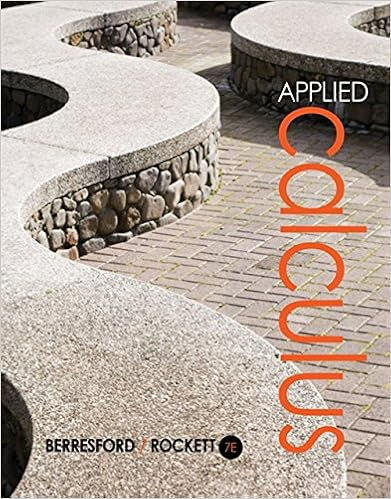# Math 1013 Tutorial 7 (Revision Exercises for Mid Term Exam)

• Test Prep
• 4

This preview shows page 1 out of 4 pages.

##### We have textbook solutions for you!
The document you are viewing contains questions related to this textbook.The document you are viewing contains questions related to this textbook.
Chapter 3 / Exercise 74
Applied Calculus
Berresford/RockettExpert VerifiedUnformatted text preview: (T8) Math 1013 Tutorial 7 (Revision Exercises for Mid Term Exam) (1) Page | 1 Derivative of the Inverse Function 1) Evaluation of Composite Functions If () = √ and () = − , , find the compositions 2) , . Domain of a Function Find the Domain and Range of the following Functions : (a) () = ( − )√ + (b) ) = ( − ) + (c) () = (+)(−) (d) () = √ − (e) () = Example 1 − √+ 3) State the condition (criteria) that a function () has an inverse function − (). 4) Suppose that −− () = { − ≠ = (a) Find → (). (b) Is () continuous at = ? (c) Is () differentiable at = ? (T8) Math 1013 Tutorial 7 (Revision Exercises for Mid Term Exam) 5) () = − Evaluate →∞ () and →−∞ () for 8) √ − (a) Find →− (). (a) (b) Find →+ (). (b) (c) 6) √ + () = () = ( − √ + ) What is the value of () so that () is continuous at = . Why? Evaluate the Limits : (a) → (b) → √++ () = { − + < = ≥ Determine values of the constants and for which g is − + continuous at = . (−)√+ 10) Determining the unknown constant −√+ →∞ ( + (e) →−∞ √ () = { ) − + − ≤ > For what value of a for which ′() exist 11) Find √ + + + = ( + ) (c) = (( )) () = {√ − = , if : (b) (d) = (√ ) (f) = ( + ) (g) = ( + + ) (a) →+ ( ) If () is definded by 9) (d) (f) +√ + → (c) 7) Page | 2 ≤ − < < ≥ (h) Compute the following limits or state that they do not exist. (a) →−− () (b) →− (d) →− () (e) →+ () (f) → (i) () = (+) / (−) / (+) / →−+ () (c) () = () () 12) Prove that → and → do not exist. (T8) Math 1013 Tutorial 7 (Revision Exercises for Mid Term Exam) 13) ′ () = , () = , ′ () = , Given that () = , ′ () () = , (a) If () = 18) (a) Suppose the slope of the curve = . () Page | 3 = − () at (4, 7) is , find ′() . () (b) Find (− )′() if () (b) If () = ()()() , find ′() . . Find ′() . () = + + . If () = (()) , find ′(). (c) 19) Inverse functions (d) 14) If () = (() )(() ) √() Find the inverse functions and the derivatives of their inverse functions for the following functions : , find ′(). Find the equations of tangents to the following curves at the given points : + − (b) () = + () = | + | for ≥ − . = ; (, ) + (b) + = ; (, ) (c) ( + − ) = ( + ) ; (2, 2) (d) √ + = + , (1, 0) 15) () = √ + , Find ′() and ′(). 16) Find (a) (c) (e) 17) () = (c) (a) (a) ( + ) ( + ) ( ) (b) (d) ( + ) (( ) ) Find the derivates of the following functions : (a) () = − ( ) (b) () = − ( + ) (c) () = − √ (d) () = (− ) 20) Rate of Change Math 1013 Tutorial 7 (Revision Exercises for Mid Term Exam) 21) 23) 22) 24 (T8) Page | 4 ...
View Full Document

•••